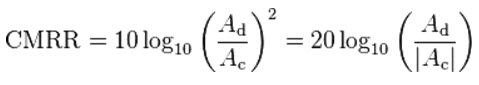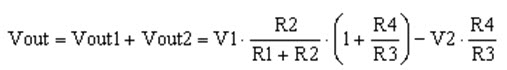# What is Differential Amplifier Circuit and Equation

Thus far away we have got used only one of the op-amp i/ps to connect to the amplifier. The op-amp ‘s two inputs is named as inverting or non-inverting terminal. These terminals are used to amplify one i/p with the opposed input being connected to ground. However, we are able to conjointly connect signals to each of the inputs at the same time designing another common form of op-amp circuit which is called as a differential amplifier. It is basically used as a building block of an operational amplifier which is called as operational amplifier (op-amp). The main function of the differential amplifier is, it amplifies the changes between two i/p voltages. But, conquers any voltage common to the two i/ps. This article gives an overview of differential amplifier along with its mathematical expressions.

## What is a Differential Amplifier

All operational amplifiers (op-amps) are differential amplifiers because of their input configuration. When the first voltage signal is connected to the input terminal and another voltage signal is connected onto the opposite input terminal then the resultant output voltage are proportional to the difference between the two input voltage signals of V1 and V2. The output voltage can be solved by connecting each i/p intern to 0v ground using super position theorem.

## Op-Amp as a Differential Amplifier

An op-amp is a differential amplifier  which has a high i/p impedance, high differential-mode gain, and low o/p impedance. When the negative feedback is applied to this circuit, expected and stable gain can be built. Usually, some types of differential amplifier comprise various simpler differential amplifiers. For instance, a fully differential amplifier, instrumentation amplifiers and an isolation amplifier are frequently built for various operational amplifiers.

• Differential amplifier is used as a series negative feedback circuit by using an op-amp
• Usually, differential amplifier is used as a volume and automatic gain control circuit
• Some of the differential amplifiers can be used for AM (amplitude modulation).

Internally, here are many electronic devices uses differential amplifiers. The ideal differential amplifier o/p is given by

In the above equation, A is the differential gain and Vin+ and Vin-  are the i/p voltages. In practice, the gain is not equal for the inputs. For example, if the two i/p voltages are equal, then the o/p will not be zero, A more accurate expression for a differential amplifier comprises a second term.

In the above equation “Ac” is the common mode gain of the differential amplifier. When these amplifiers are frequently used to bias voltages or null out noise that appear at both i/ps., a low common mode gain is usually desired.

The CMRR is nothing but common mode rejection ratio, the definition of the MMR is, it is the ratio b/n differential mode gain &a  common mode gain, specifies the capacity of the amplifier to exactly cancel voltages that are common to both i/ps. The CMMR is defined asIn an ideal differential amplifier, Ac is  zero and (CMRR) is infinite.

### Differential Amplifier Transfer Function Calculation

The T/F of the differential amplifier is also called as difference amplifier, and the transfer function of the differential amplifier equation is shown below

Vout=v1.R2/R1+R2(1+R4/R3)-V2.R4/R3

The above formula relates only for an idle operational amplifier  which has a large gain (considered as infinite) and the i/p offset is small ( considered as zero). For instance, in the following circuit the i/p voltage levels are around a few volts and the input offset of the op-amp is millivolts, then we can consider it as zero by neglecting the i/p offset.

The transfer function of the differential amplifier is derived from the superposition theorem, which states that,  in a linear circuit the effect of all sources is the algebraic sum of the effects of each source taken individually. In the above circuit, when we remove V1 and short circuit it, then the o/p voltage will be calculated. In the same manner remove V2. The differential amplifier’s o/p voltage is the sum of the both the o/p voltages.

Lets remove R1 and V1 in the circuit below. Because in the first circuit there was a flow of current through it. So, ground the resistor R1. When we observe the circuit, it becomes an inverter. This circuit noninverting i/p terminal is connected to the ground terminal through resistors R1 and R2. Then the Vout is
Vout2=-V2. (R4/R3)
Now let’s ground R3 and remove V2 shown in the circuit below.

This circuit is a non inverting amplifier, and for an ideal op-amp, Vout is a function of V, that is the voltage connected to ground at the non inverting terminal of the op-amp
Vout1=V. (1+R4/R3)
The R1, R2 resistors is an attenuator for V1, so the V can be determined as in the following equation.
V=V1.R2/R1+R2

By substituting equation V in the equation of Vout, then it becomes
Vout1=V1.R2/R1+R2.(1+R4/R3)

Now we have Vout1 and Vout2, according to the superposition theorem Vout is the sum of Vout1 & Vout2The above equation id the transfer function of the differential amplifier.

#### Differential Amplifier using Wheatstone Bridge

The typical Differential Amplifier circuit now becomes a differential voltage comparator by “Comparing” one i/p voltage to another. Here, for instance, one input is connected to a fixed voltage reference set up on one leg of the resistive bridge n/w and another input to either a “Light Dependant Resistor” or “Thermistor”. The amplifier circuit is used to detect either low or high temperature levels or light as the o/p voltage becomes a linear function of the changes in the active leg of the resistive bridge.